# Calculating N-Spheres

As a result of my years studying String Theory at the undergraduate level, I eventually developed a method for determining the properties of N-Spheres, with the ambition and then-enthusiastic hope of finding the required testing distance over which the strength of gravity would need to be measured to determine the number of physical dimensions there are in space.  While I did eventually come up with an equality that might produce an experimental setup, the choices I made with my career after my undergraduate degree obviated the need for further investigation.  In any event, I thought I would share what original research I did develop, so here is goes.

Why Spheres?

Gravity is weak.  This is well known and endlessly recited, but the explanations for why it might be weak are often too esoteric to delve into.  One proposal  comes out of string theory research (now several decades old), and revolves around the properties of the graviton.  Although presently undetected, the graviton is a proposed member of the complete standard model, and serves as the carrier of the force of gravity.  These particles are theoretically exchanged by massive objects and thereby guide their attraction to one another.

But why is the strength of gravity so far out of whack from the other forces?  Employing an example of this weakness from Brian Green, think about a common refrigerator magnet.  Here we have a competition — a battle of strength against a few ounces of iron on the magnet, and the gravitation pull of the entire planet Earth.  Were gravity and electromagnetism on equal footing, the magnet would fall straight to the ground (and weight much more than a few ounces for that matter).  But that is not what we actually see.  In fact, the few ounces of iron have no trouble at all resisting the pull of the Earth’s gravity, and the magnet sticks resolutely to your refrigerator.

In string theory, there are more than three dimensions in real space, but those extra degrees of freedom are only accessible to certain types of string configurations, one of which is the graviton, and only over very small distance scales.  In normal 3-space, a force emanating from a point will expand as a spherical wave, thereby experiencing a flux density that drops in proportion to R squared.  Why squared?  Because the total flux emitted by this point must spread itself over the surface of a sphere growing  with time, therefore the density is the total flux divided by the surface area of a sphere.  This can be clearly seen built into the common equations of force.  Take for instance Gravitation: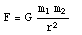‘G’ is the gravitation constant, and being a constant it can accurately be rewritten as any combination of constants, leading to the unusual but entirely accurate formulation: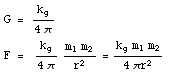And there we have it, a flux (numerator), divided over the surface area of a sphere.  This is visible again in Coulomb’s Law, and here the constant is often defined in terms of 4π:Again the flux (numerator) is divided by the surface of a three-dimensional sphere.  So if you are dealing with these forces, you are dealing with spheres, and if you are dealing with three dimensions, you are dealing with ordinary looking “2-spheres” described by their usual properties.

Moving to N-Sphere

Without going into too much detail, the string theory explanation is pretty simple.  If string theory is correct about the true makeup of space-time, then over very small distances the force of gravity is much stronger.  As gravitons emit from a source, many of them drift off into extra dimensions.  The pieces that stay in our coordinates then move along and obey normal 2-sphere propagation attenuation, but appear as if they were very weak to begin with, as so many gravitons have already disappeared.  What I sought to accomplish was to determine what the force of gravity should actually look like in small scales, if space-time has more than three dimensions.  Clearly a 2-Sphere no longer describes the real “surface” that the net flux is distributed over.  The theoretical test, once a n-sphere formulation of gravity is identified, would be to find a means of balancing gravity against electromagnetism (a force that cannot see the extra dimensions, and is therefore 2-spherical all they way down) and see where they balance.

So one last time, the gravitational FLUX is described by the numerator in the equation above, and we are searching for the new surface to distribute it over.  Let psi-g represent the flux, and the constant k-g will be defined as follows (or merely the gravitational constant divided by 4π):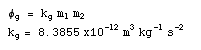Spheres

When trying to imagine N-Spheres, like many before me, I work on analogy starting from lower dimensions.  Lets bear in mind the definition of a circle (a 1-Sphere) to help extrapolate: the set of all points equal distance from one center point.  In the case of a 0-Sphere, (a “sphere” that exists on a single line), the “set of all points” would refer to only two points.  The point R away from me along the line in one direction, and the point R away from me along the line in the other direction.  The “volume” of this sphere can be calculated by doing a “shadow integral” over an identity function running between R and -R.  As with any sphere, the surface area is then the derivative of the volume.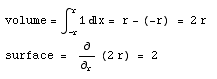These answers make some sense intuitively.  If you have two points, separated by 2r then the “volume” of your 1-d enclosure is simply the length of line between them, or 2r.  The “surface” is really the sum of two points, which is harder to visualize, but expressed in the math.  Lets move ahead to 1-Spheres… commonly called circles.  We already know what to expect from these results, but lets test it.  Now I am going to do a “shadow” integral over an identity function, first describing a circle, and then from -r to r once again: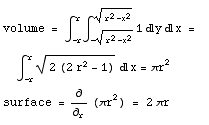Lets do the last familiar case, so the pattern in the equation can emerge.  Here is the 2-Sphere: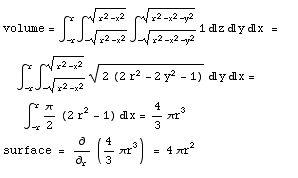Whenever you want to determine the “volume” of the next dimensional sphere, you need to first integrate over a circle that contains all of the degrees of freedom in question, and then treat that as your “shadow” and integrate up each dimension in the chain.  As expected, each dimension adds an extra power to the radius, which each time extends into a new degree of freedom.  Here are the results of the next several N-Spheres, arriving ultimately at a 9-Sphere whose surface can describe the flux distribution in an 11-dimensional string theory (special thanks to the TI-89 Titanium, and several sets of batteries!):Here are the final order ones that matter for String theory.  These took hours to verify on my TI-89, although I originally determined these using an expansion I derived from the previous 5.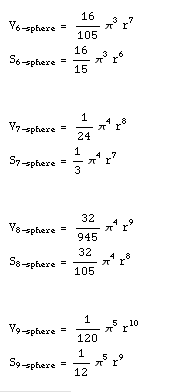And there we have it.  String theory calls for at least 11 dimensions, meaning one time dimension and 10-spatial dimensions.  A ten dimensional sphere, or a 9-Sphere (9 because the numbering indicates the number of dimensions that makeup the bounding surface), therefore describes the needed volume and surface.  Of course I make no claim of having inventing the concept of shadow integrals, but I can take credit for deciding to use them to solve for N-Spheres in this way.  Just to cap this off, here is my formula (fully original, deduced only from my previous calculations) for finding the volume of an N-Sphere.  I have not proven this formula mathematically, but I have verified its predictions up to 10-Spheres: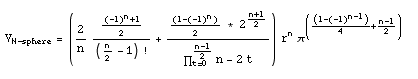New equivalence

If string theory is correct and gravity is very strong on a small scale, the relative strength of gravity compared to electromagnetism should change drastically on those scales.  I surmised that a test could be undertaken, attempting to find an equilibrium between the attractive force an electron’s gravity, and its repulsive force of its electromagnetism.  Let see on what scale such an equilibrium could be found: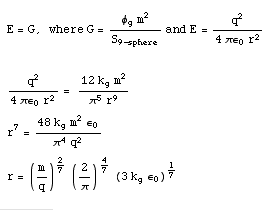Now we can solve this equation with the known values for an electron, and we find: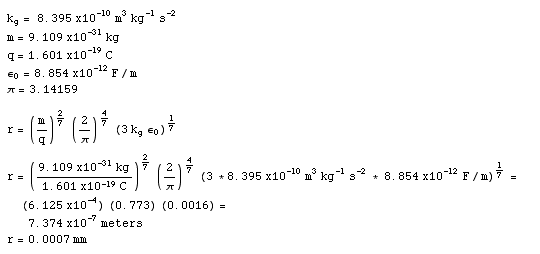So when two electrons are brought to a halt about one thousandth of a millimeter from one another, we should be able to get them to balance IF a) string theory’s proposition of 11-dimensions is correct, and 2) if the wrapped up dimensions described in the theory are on the order of 1000ths-of-millimeters or more.

In other formulations of string theory that require more dimensions, this equilibrium shrinks.  Unfortunately, current experiments have only been able to probe the strength of gravity on scales two orders of magnitude greater (tenths of millimeters), which suggests an answer will not be forthcoming for at least a couple years.  But in any case, lack of any finding is insufficient to falsify string theory because either of the two premises leading to the above equivalence may be wrong without string theory itself necessarily being wrong.

Avoiding the slippery slope that is my personal dislike of string theory, let me leave this entry alone as only a commentary on N-Spheres.  Well, now I can’t say my undergraduate degree in physics was a complete waist!  At least I got one Blog entry out of it.